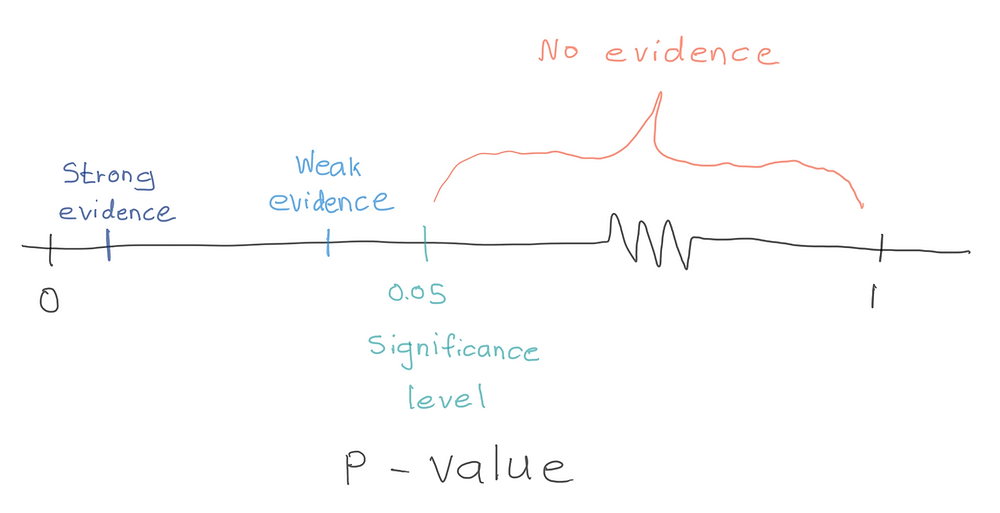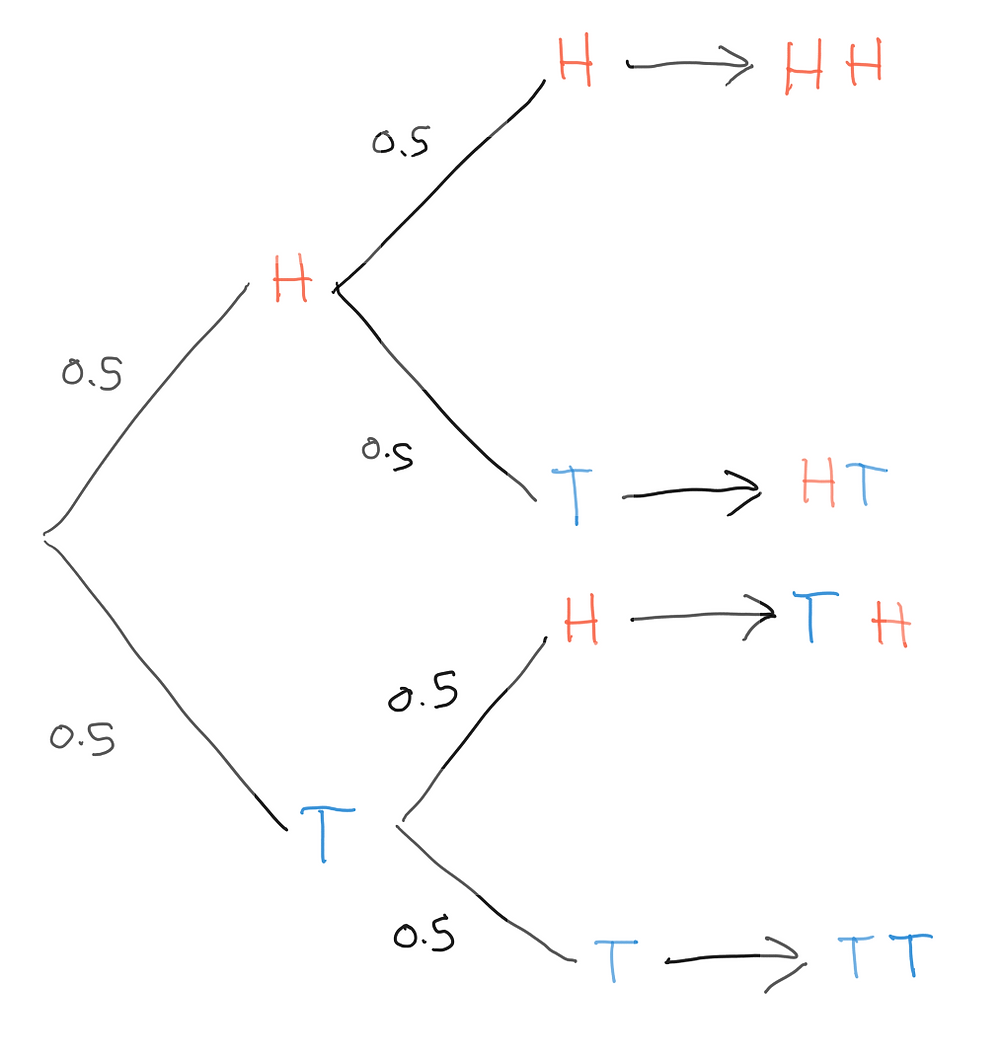Search
•Savidu Dias

# To Noobs with Love: Machine Learning: P-Values and Significance Tests

Updated: Mar 27, 2020

Before I get into the really fun stuff, I want to get something really important out of the way. This is something that took me days to understand, which is P-Values and Significance tests. Before we begin, let’s get some terminology out of the way.

## Null Hypothesis

Null Hypothesis is used in statistics to propose that there is no difference between certain characteristics of a set of data. What does this mean? Let’s look at it with a simple example. A manufacturer of a certain hand sanitizer claims that their hand sanitizer contains 60% alcohol.The null hypothesis is that the alcohol percentage of a bottle of hand sanitizer is 60%.In order to test this claim, we can’t take all of their bottles of hand sanitizer (for obvious reasons). Instead, we take a sample of 30 bottles from the entire population and calculate the mean alcohol percentage of that sample.

We can then compare the calculated sample mean to the claimed population mean of 60% and attempt to reject the null hypothesis. Analysts look to reject the null hypothesis.

## Alternate Hypothesis

In statistics, we are interested in proving whether a working statement (the null hypothesis) is true or false. Usually, these are expected to be true — some kind of historical or existing expected value.The alternate hypothesis is simply an alternative to the null. In our null hypothesis example above, the alternate hypothesis would be that the average alcohol percentage is less than 60%.

In many cases, the alternate hypothesis will be the opposite of the null hypothesis.

## Significance Level

The significance level is the probability of rejecting the null hypothesis when it is true. For example, a significance level of 0.05 indicates a 5% risk of concluding that a difference exists when there is no actual difference. Still confused? Let’s look at it visually.In the graph above, the sum of bottles having alcohol levels below x1 and above x2 represent 5% of the population. The two shaded regions have an equal distance from the null hypothesis of 60%. Each area has a probability of 0.025.

Since the population mean is 60, we would expect to obtain a sample mean that falls in the shaded area 5% of the time.

If our mean sample alcohol percentage of 58% is less than x1, we can say that it is statistically significant at the 0.05 level

In this case, we can say that the CEO of Hand Sanitizer Inc is a big ol’ liar. This is called rejecting the null hypothesis.

Conversely, if the sample percentage of 58% is more than x1, we do not have enough evidence to reject the null hypothesis.

## P-Value

In statistics, p-value is the probability of obtaining results as extreme as the results observed in the sample test. This assumes that the null hypothesis is correct.

For example, if the mean alcohol percentage of the hand sanitizer sample we took earlier was 58%, the p-value is a measurement used to determine the probability of getting a mean alcohol percentage of 58% or lower from a sample if the average alcohol percentage is 60% or more.

If the p-value is less than the significance level, our original idea must have been wrong. Therefore, we have enough evidence to reject the null hypothesis.

A small p-value indicates a significant result. If the p-value is large, then our original idea is probably correct. Then we do not reject the null hypothesis. This is called a non-significant result.## Calculating the P-Value

Let’s look at a simple example to get a basic idea on how to calculate a p-value.

Consider a situation where we flip a coin two times. What is the probability of getting two heads in a row, and what is the p-value for getting 2 heads in a row?In this example, there are 4 different outcomes, each of which are equally likely. Therefore, the probability of getting two heads in a row is calculated as follows.What about 1 head and 1 tails? The probability of getting one H and one T regardless of order is:

2/4 = 0.5

When calculating the probability, order of things does not matter.

What is the p-value for getting two heads in a row?

A p-value is the probability that random chance generated the data, or something that is equal or rarer.

A p-value consists of 3 parts:

1. The probability that random chance generated the data you observed

2. Anything else in the outcome that has equal probability

3. Anything that is rarer than what was observed

When calculating the p-value for 2 heads in a row:

1. The probability that random chance generated two heads is ¼ = 0.25

2. Getting TT from 2 coin flips has an equal probability of ¼ = 0.25

3. There are no outcomes rarer than getting HH.

P-Value for HH = 0.25 + 0.25 + 0 = 0.5

The probability of getting HH is 0.25 The p-value for getting HH is 0.5

Now let’s look at a slightly more complicated example.

This time, we will be flipping the coin 5 times. Shown below are all possible outcomes of the coin tossesFrom these outcomes, we can see that:

• There are 32 possible outcomes.

• There is 1 outcome where all heads are observed.

• There are 5 outcomes where 4 heads and 1 tail is observed.

• There are 10 outcomes where 3 heads and 2 tails are observed.

• There are 10 outcomes where 2 heads and 3 tails are observed.

• There are 5 outcomes where 1 head and 4 tails are observed.

• There is 1 outcome where all tails are observed.

The probability of getting all heads is 1/32 = 0.03125

When calculating the p-value for getting 5 heads in a row:

1. The probability that a random chance generated 5 heads is 0.03125.

2. Getting 5 tails has an equal probability of 0.03125.

3. There are no outcomes rarer than getting 5 heads.

P-Value for HHHHH = 0.03125 + 0.03125 + 0 = 0.0625

When calculating the p-value for getting 4 heads and 1 tails:

1. Probability that a random chance generated 4H and 1T is 5/32 = 0.15625

2. Getting 1H and 4T has an equal probability of 5/32 = 0.15625

3. Probability of 5H or 5T are rarer outcomes

P-Value for 4 heads 1 tails = P(4H 1T) + P(1H + 4T) + (P(5H) + P(5T)) = 0.375

Let’s get back to our hand sanitizer example.This is called a density. The area under the curve indicates the probability that a person will have a height within a range of possible values. 95% of the area under the curve is between 58 and 62 indicating that most bottles of hand sanitizer have alcohol levels between those values. That is, there’s a 95% probability that each time we measure the alcohol percentage in a bottle of hand sanitizer, it will have a value between 58 and 62 percent.2.5% of the total area of the curve is greater than 62, and 2.5% of the area under the curve is less than 58.

To calculate p-values, you add up the percentages of areas under the curve. For example, the p-value for a bottle of hand sanitizer with an alcohol level of 58% is:

1. 2.5% for the bottles with a level of 58 percent or lower.

2. 2.5% of the bottles with a level of 62 percent or higher.

These values account for the “equal or rarer” part of calculating a p-value.

Therefore, the p-value is 0.025 + 0.025 = 0.05

According to this density graph, our p-value is equal to the significance level. Therefore, we have enough evidence to reject the null hypothesis.

We now have incriminating evidence against the CEO of Hand Sanitizer Inc. and he will spend the rest of his life behind bars thanks to p-value.Congratulations! Your knowledge in statistics has led the CEO of Hand Sanitizer Inc. to be found guilty of fraud. Let’s put your knowledge to the test and calculate the p-value for a bottle that has an alcohol percentage between 59.7% and 60.3%.• The probability of a bottle having an alcohol level between 59.7% and 60.3% was calculated to be 0.04 (4%).

• Note that any other alcohol percentage is rarer than this probability. That is the probability of a rarer observation is 0.96% (96%).

Therefore, p-value for a bottle that has an alcohol percentage between 59.7% and 60.3% is

p-value = 0.04 + 0.96 = 1

That means there’s nothing special about measuring a bottle that has an average alcohol percentage. In this example, the probability of measuring a container within this range is tiny (0.04), but the p-value is huge (1).

Congratulations! You have mastered p-values and significance tests. You deserve a pat in the back. Go grab a snack or something. BYE.